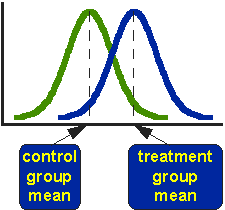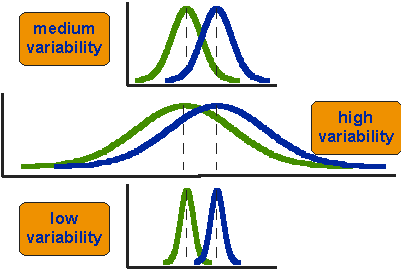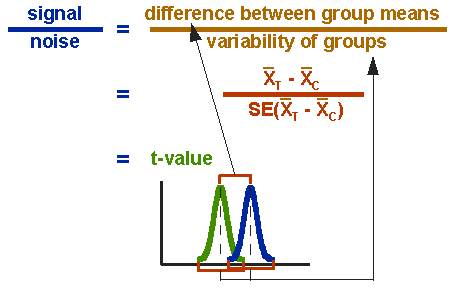The T-Test

# The T-Test

The t-test assesses whether the means of two groups are statistically different from each other. This analysis is appropriate whenever you want to compare the means of two groups, and especially appropriate as the analysis for the posttest-only two-group randomized experimental design.Figure 1. Idealized distributions for treated and comparison group posttest values.

Figure 1 shows the distributions for the treated (blue) and control (green) groups in a study. Actually, the figure shows the idealized distribution – the actual distribution would usually be depicted with a histogram or bar graph. The figure indicates where the control and treatment group means are located. The question the t-test addresses is whether the means are statistically different.

What does it mean to say that the averages for two groups are statistically different? Consider the three situations shown in Figure 2. The first thing to notice about the three situations is that the difference between the means is the same in all three. But, you should also notice that the three situations don’t look the same – they tell very different stories. The top example shows a case with moderate variability of scores within each group. The second situation shows the high variability case. the third shows the case with low variability. Clearly, we would conclude that the two groups appear most different or distinct in the bottom or low-variability case. Why? Because there is relatively little overlap between the two bell-shaped curves. In the high variability case, the group difference appears least striking because the two bell-shaped distributions overlap so much.Figure 2. Three scenarios for differences between means.

This leads us to a very important conclusion: when we are looking at the differences between scores for two groups, we have to judge the difference between their means relative to the spread or variability of their scores. The t-test does just this.

## Statistical Analysis of the t-test

The formula for the t-test is a ratio. The top part of the ratio is just the difference between the two means or averages. The bottom part is a measure of the variability or dispersion of the scores. This formula is essentially another example of the signal-to-noise metaphor in research: the difference between the means is the signal that, in this case, we think our program or treatment introduced into the data; the bottom part of the formula is a measure of variability that is essentially noise that may make it harder to see the group difference. Figure 3 shows the formula for the t-test and how the numerator and denominator are related to the distributions.Figure 3. Formula for the t-test.

The top part of the formula is easy to compute – just find the difference between the means. The bottom part is called the standard error of the difference. To compute it, we take the variance for each group and divide it by the number of people in that group. We add these two values and then take their square root. The specific formula for the standard error of the difference between the means is:

$$\textrm{SE}(\bar{X}_T-\bar{X}_C) = \sqrt{\frac{\textrm{var}_T}{n_T}+\frac{\textrm{var}_C}{n_C}}$$

Remember, that the variance is simply the square of the standard deviation.

The final formula for the t-test is:

$$t = \frac{\bar{X}_T-\bar{X}_C}{\sqrt{\frac{\textrm{var}_T}{n_T}+\frac{\textrm{var}_C}{n_C}}}$$

The t-value will be positive if the first mean is larger than the second and negative if it is smaller. Once you compute the t-value you have to look it up in a table of significance to test whether the ratio is large enough to say that the difference between the groups is not likely to have been a chance finding. To test the significance, you need to set a risk level (called the alpha level). In most social research, the “rule of thumb” is to set the alpha level at .05. This means that five times out of a hundred you would find a statistically significant difference between the means even if there was none (i.e., by “chance”). You also need to determine the degrees of freedom (df) for the test. In the t-test, the degrees of freedom is the sum of the persons in both groups minus 2. Given the alpha level, the df, and the t-value, you can look the t-value up in a standard table of significance (available as an appendix in the back of most statistics texts) to determine whether the t-value is large enough to be significant. If it is, you can conclude that the difference between the means for the two groups is different (even given the variability). Fortunately, statistical computer programs routinely print the significance test results and save you the trouble of looking them up in a table.

The t-test, one-way Analysis of Variance (ANOVA) and a form of regression analysis are mathematically equivalent (see the statistical analysis of the posttest-only randomized experimental design) and would yield identical results.